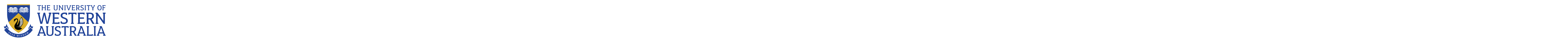School of Mathematics and Statistics

# School of Mathematics and Statistics

Research being undertaken by our current postgraduates:

Donny Lesmana

Sep 2009

### Donny Lesmana

#### Thesis

Numerical Methods for Solving Black-Scholes Option Pricing Equations with Transaction Cost

#### Summary

Based on the studies of computational theories and algorithms of stochastic control and optimal stopping, I will develop efficient and accurate methods for calculating numerically the prices of European and American pricing options with proportional costs both when the underlying asset prices follow geometric Brownian motion and jump diffusion process, which are in the form on nonlinear partial differential equations. The research will further develop the nonlinear Black-Scholes option pricing model.

#### Why my research is important

The results of this study will form a basis for future research in the area of nonlinear option pricing models.

The Black-Scholes model is a very effective method for option pricing. However, in the presence of transaction costs on trading in the riskless security or stock, the Black-Scholes option pricing methodology is no longer valid, since perfect hedging is impossible. The constant rebalancing used in the Black-Scholes framework will be infinitely costly, no matter how small the transaction costs are, since the geometric Brownian motion has infinite variation.

Based on the studies of computational theories and algorithms of stochastic control and optimal stopping, I will develop efficient and accurate methods for calculating numerically the prices of European and American options with proportional costs both when the underlying asset prices follow geometric Brownian motion and jump diffusion process, which are in the form of nonlinear partial differential equations. The research will further develop the nonlinear Black Scholes option pricing model. The results of this study will form a basis for future research in the area of nonlinear option pricing model.

### Statistics clinic

Assistance in statistics is available for Postgraduates students by research at the UWA Centre for Applied Statistics.

# The University of Western Australia

## University information

CRICOS Code: 00126G